Determining the Material of a Ferrite Core

# Determining the Material of a Ferrite Core

The main characteristic differentiating Fair-Rite’s material grades is initial permeability. This is the inductive portion of the complex permeability of a material measured specifically at 10kHz with a flux density of less than 10 gauss (sinusoidal excitation). Calculating the initial permeability on the sample in question is the most reliable way to get an approximation of what material it is made of. To evaluate the permeability, you will need insulated wire and some way to measure inductance with a 10kHz sinusoidal signal (in a pinch 1kHz-100kHz should be about the same for most materials). In general, an LCR meter is the optimal piece of equipment to make this measurement. With a bit of extra effort, a signal source along with an oscilloscope could be used. By far the easiest geometry to evaluate would be a toroidal type shape though any core with a closed magnetic structure can be evaluated with a reasonably high degree of accuracy. Cores with air gaps in the structure can be evaluated as well though the ability to differentiate between material grades is reduced as the length of the gap increases. “Open” magnetic structures can be evaluated for permeability as well though the ability to differentiate between different material grades depends heavily on the dimensions of the core and the winding applied.

###### Closed Magnetic Structures

The first step in evaluating initial permeability in a closed magnetic structure is to measure the main physical dimensions of the core. The goal here is to calculate core parameter C1 (cm-1) which is the effective path length (∑l) divided by the cross-sectional area (Ae). This parameter is needed to calculate air core inductance denoted as L0 (H) for the core in question. The equation to calculate L0 is as follows: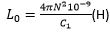In this equation N=the number of turns on the core. Toroidal type cores are the easiest to calculate due to their uniform cross-sectional area, circular path length, and lack of air gap.

Once the L0 for the core has been calculated, the inductance must be measured. Multiple turn windings should be used to mitigate the significance of the inductance added by the WIRE in the measured inductance of the core assembly. This will prevent the permeability measurement from being skewed high (especially in low permeability materials) by the inductance of the wire itself. Five to ten turns are recommended through the aperture of the core or around the center post in the case of a mated geometry or multi-aperture type core. Cores with a higher path length to cross-sectional area should have higher turn counts to mitigate the effects of the wire. The wound core should then be measured on an LCR meter set to 10kHz (1-100kHz values should be similar for most materials) and the series inductance value recorded. For LCR meters that have an adjustable oscillation level; The voltage should be adjusted so that the peak flux density (Bpk) remains below 10 gauss: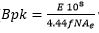where E=RMS sine wave voltage, f=Frequency in Hz, N=Number of turns, and Ae=Effective cross-sectional area in cm2. Something to keep in mind is that the permeability will be affected by a sufficiently high peak flux density. To get from inductance to initial permeability the measured Ls value in Henries should be divided by the calculated L0 value in Henries. For mated or gapped cores, the resulting number (effective permeability) will be the lower than the actual material permeability proportionate to the gap length.

###### Open Magnetic Structures

Evaluating open magnetic structures such as rods and bobbins is less clear cut than closed magnetic structures. Since most of the field is not contained within the core, the initial permeability has a greatly reduced influence on the inductance of the wound assembly. The length to diameter ratio of the core can significantly impact the ability to differentiate between materials. As seen in the graph below; Cores with a low length to diameter ratio have a very low effective permeability (µrod) that is nearly indistinguishable between material grades.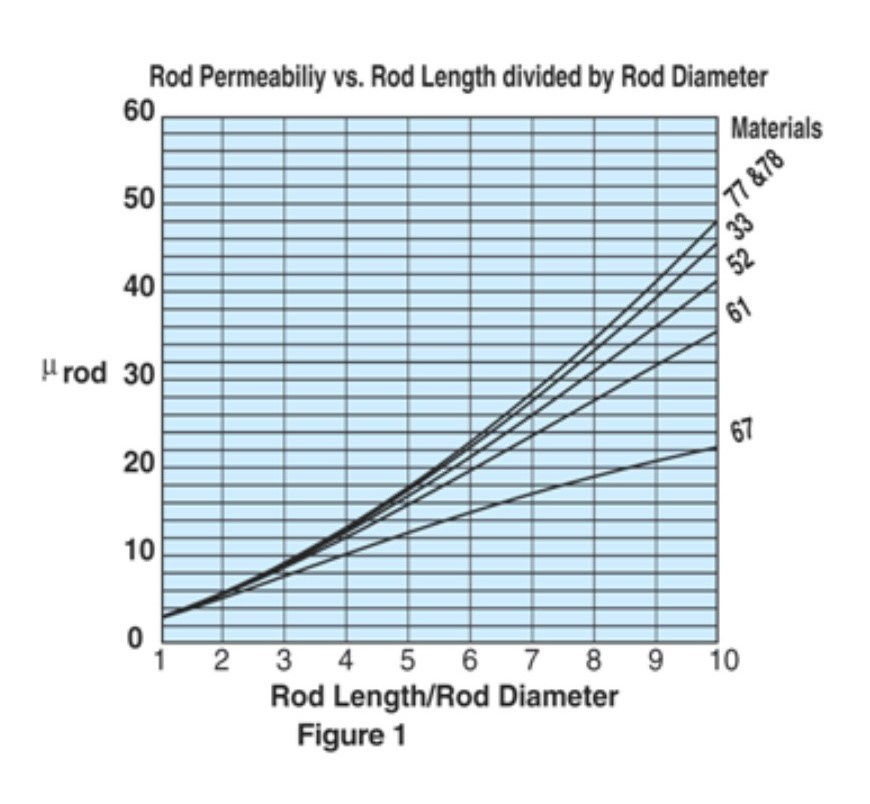A trick to evaluate a rod type core that has a low length to diameter ratio is to take several of them placed end to end and test them as one longer rod. The air gaps between the cores will have a relatively insignificant impact on the effective permeability given that most of the magnetic field is not contained within the core. This can be seen in the practical test example below where low length to diameter ratio rods in 77 & 61 material are stacked together to make a progressively longer rod. In doing so the effective permeability (indicated by the inductance) is easier to differentiate between the two materials as more rods are put together.An example of this is shown below. As can be seen in the below example; the higher permeability material (77) results in a lower inductance than the lower permeability material (61) when the L/D ratio is low. This is not genuinely indicative of the relative performance of the materials. The difference is more to do with the difficulty in measuring a low L/D ratio rod due to the relatively small influence that the materials permeability has on inductance.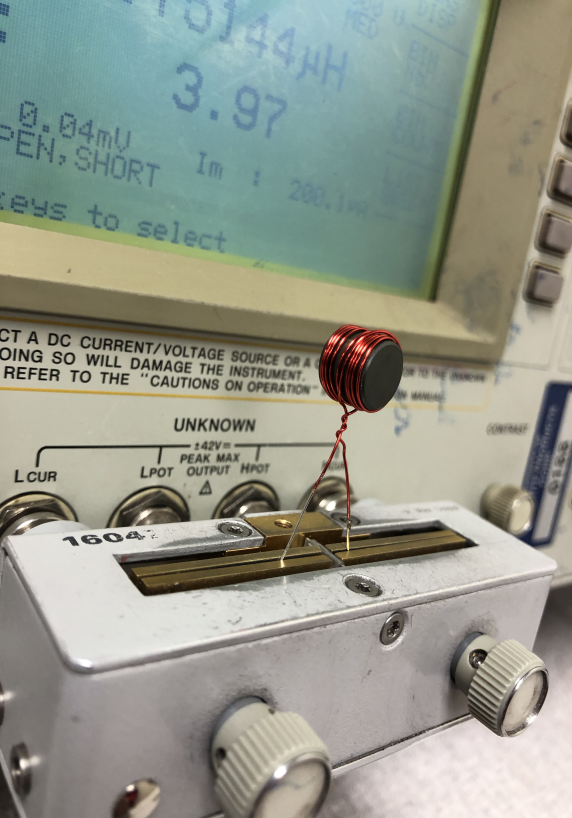Diameter Length L/D Ratio Number of Cores N Inductance 77 Material Inductance 61 Material 9.474 8.17 0.86 1 10 2.75 2.98 9.474 24.50 2.59 3 30 34.15 29.4 9.474 48.99 5.17 6 60 171.93 137.97

Coverage of a coil over a rod is an important factor as well. Getting a complete coverage of the rod will yield a lower inductance modifier (K) and therefor a higher inductance making distinguishing between different materials easier. The inductance modifier trend vs the coil length/rod length ratio can be seen below.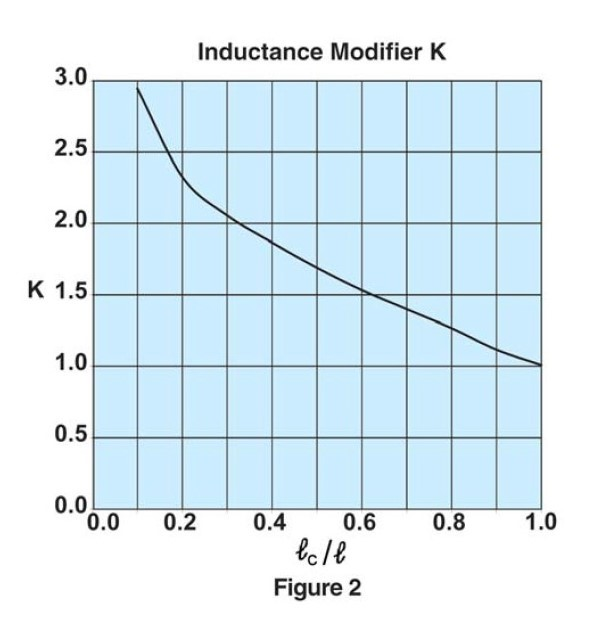With the information gathered so far, it is possible to back calculate the materials effective permeability (µrod) from this formula. where: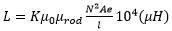K= Inductance modifier                µ0= 4π10-7µrod= Effective permeability (figure 1)      N= Number of turns Ae= Cross sectional area of the rod (cm2) L= Length of the rod (cm)           Lc= Length of the winding (cm)

Once µrod is calculated, the resulting value should be compared to the chart in figure 1 to match it to the listed materials. Materials that are not specifically listed in can be interpolated based on initial permeability relative to materials which are listed on the chart. For example, if µrod is higher than 77/78 material at a given length to diameter ratio it could be inferred that the material used has a higher initial permeability (like a 75 or 76 material) than 77 or 78 material.

With open and closed magnetic structures there is potential for some overlap of permeabilities between different materials. Often there is the possibility of a NiZn and MnZn ferrite having the same or similar permeability. An easy method for distinguishing between these two basic materials is by measuring the resistance of the material. There are specific resistance meters geared towards precisely measuring bulk resistivity but, to determine between these two basic types probing across a small area on the surface of the part with a simple multimeter will be sufficient. NiZn ferrites have a relatively high DC bulk resistivity from around 100000 ohm-cm and up. MnZn ferrites have a DC bulk resistivity of around 3000 ohm-cm and lower. While a basic multimeter might not have the range to measure NiZn ferrites an out of range high reading would indicate towards the material being NiZn rather than MnZn.

###### Conclusion

The initial permeability and rough estimation of the permeability will give a fairly good estimation of the material a ferrite core is made from. Occasionally more than one ferrite material will meet or match the two characteristics measured. To distinguish these materials; Secondary characteristics such as power losses, saturation flux density, curie temperature and many others need to be looked at to differentiate. These characteristics generally require more specialized test equipment/procedures to measure. In lieu of being able to measure these characteristics, sending a sample in to Fair-Rite for testing is always an option. Another is creating a short list of materials and trying them in-situ. It is possible that the original core material might not be the best core material for the application (it may have been at the time). It is also possible that more than on material will work fine for the application if it is not constrained by any of these secondary characteristics.

You must be signed in to use the forums